Journal of Vibroengineering

Published: 15 August 2022

# Hierarchical dispersion entropy and its application in fault diagnosis of rolling bearing

Tao Zhang1
Yongqi Chen2
Yang Chen3
1, 2, 3College of Science and Technology, Ningbo University, Ningbo, 315211, P. R. China
Corresponding Author:
Yang Chen
Views 48

#### Abstract

Aiming at the disadvantage that multi-scale entropy only analyzes the low-frequency components of time series, this paper proposes a rolling bearing fault feature extraction method based on hierarchical dispersion entropy (HDE). Firstly, the HDE is constructed based on the hierarchical operators and dispersion entropy. Secondly, the influence of HDE parameters on entropy stability is studied. Finally, the fault diagnosis method based on HDE, least square support vector machine is applied in rolling bearings. The experiment results show that the proposed method can distinguish different types of the rolling bearing faults. It owns the higher fault diagnosis accuracy than the traditional multi scale entropy methods, such as multi-scale sample entropy (MSE), multi-scale dispersion entropy (MDE).

#### Highlights

• HDE is used to extract rolling bearing fault features.
• HDE is constructed based on the hierarchical operators and dispersion entropy.
• HDE and LSSVM are applied into rolling bearing fault diagnosis system. It can distinguish different fault types with a higher accuracy than traditional methods.

## 1. Introduction

As one of the key parts of rotating machinery, the running health of rolling bearing is related to the operation of the whole equipment. It is of great significance to accurately diagnose the fault state of rolling bearing. Due to the complexity of working conditions, fault vibration signals often show non-stationary and nonlinear characteristics [1, 2]. Entropy is an important method to extract features of non-stationary signal. Yan et al.  put forward a machine health detection method based approximate entropy. The experiment proves this method owns a nice computational efficiency in the bearing fault diagnosis. Richman et al.  proposed sample entropy on the basis of approximate entropy, which overcomes the shortcomings of the approximate entropy self-matching. Yan et al.  applied permutation entropy to the feature extraction and condition monitoring of vibration signals. The results show that permutation entropy can detect the dynamic changes of vibration signals of rolling bearings under different conditions. However, approximate entropy, sample entropy and permutation entropy can only measure the complexity of time series on a single scale. Multi-scale entropy own multi dimensional analysis ability and strong stability, so multi-scale entropy can obtain more fault feature information and reflect the complexity characteristics. For this reason, Costa et al.  put forward the concept of multi-scale entropy (MSE). Firstly, the time series is segmented on a spatial scale, and then the entropy of samples under each scale are calculated. The complexity of time series at different scales is obtained. For this reason, more information can be analyzed by introducing multi-scale entropy. Zheng et al.  designed a fault diagnosis method of rolling bearing based on the multi-scale permutation entropy, which is applied to the fault feature extraction and diagnosis of rolling bearing. The effectiveness and superiority of the proposed method are verified by the comparison results. However, the multi-scale entropy only considers the low-frequency component of the original sequence and ignores the high-frequency component. For the rolling bearing time series with rich fault information distribution, the multi-scale entropy can't meet the requirements . In order to extract the fault information of high-frequency components in the signal, Jiang et al.  introduced the concept of hierarchical entropy. Compared with multi-scale entropy, hierarchical entropy considers both low-frequency components and high-frequency components in the signal, so as to provide more comprehensive and accurate time mode information . Azami et al. [11, 12] proposed dispersion entropy to alleviate the shortcomings of sample entropy. Compared with sample entropy, discrete entropy solves the problem of abrupt change in similarity measurement of sample entropy. Moreover, dispersion entropy has the advantages of simple and fast calculation [13-15]. Therefore, on the basis of hierarchical entropy and dispersion entropy, this paper proposes a rolling bearing fault diagnosis method based on HDE. This method can consider the high-frequency and low-frequency components of rolling bearing, and can comprehensively and accurately reflect the working state information of rolling bearing.

This paper is organized as follows: Selection 2 proposes HDE. Section 3 studies parameters selection of HDE method. Section 4 analyzes the classification results of LSSVM  based on HDE as well as the comparison results with other methods. Finally, Section 5 concludes this research.

## 2. HDE method

Based on the advantages of hierarchical entropy and the definition of dispersion entropy, HDE is proposed. The calculation process of HDE is presented as follows:

1) Given the time series $\left\{u\left(i\right),i=1,2,...,N\right\}$, the hierarchical operator ${Q}_{0}$ and ${Q}_{1}$ is defined as:

1

where $N={2}^{n}$ is a positive integer, the length of the operator ${Q}_{0}$ and the operator ${Q}_{1}$ is ${2}^{n-1}$. According to the operator ${Q}_{0}$ and ${Q}_{1}$, the original sequence can be reconstructed as:

2

When $j=0$ or $j=1$, one can define the matrix operator ${Q}_{j}$ as:

3
${Q}_{j}\left(u\right)={\left[\begin{array}{lllllll}\frac{1}{2}& {\left(-\frac{1}{2}\right)}^{j}& 0& 0& \cdots & 0& 0\\ 0& 0& \frac{1}{2}& {\left(-\frac{1}{2}\right)}^{j}& \cdots & 0& 0\\ ⋮& ⋮& ⋮& ⋮& ⋮& ⋮& ⋮\\ 0& 0& 0& 0& \cdots & \frac{1}{2}& {\left(-\frac{1}{2}\right)}^{j}\end{array}\right]}_{{2}^{n-1}×{2}^{n}}.$

(2) Construct a dimension vector $\left[{\gamma }_{1},{\gamma }_{2},...,{\gamma }_{n}\right]\in \left\{0,1\right\}$, then the integer $e$can be expressed as $\left[{\gamma }_{1},{\gamma }_{2},...,{\gamma }_{n}\right]$, where the vector corresponding to a positive integer $e$.

(3) Based on the vector $\left[{\gamma }_{1},{\gamma }_{2},...,{\gamma }_{n}\right]$, the node component of each layer decomposition of the time series $u\left(i\right)$ is defined as:

4
${u}_{k,e}={Q}_{{\gamma }_{k}}×{Q}_{{\gamma }_{k-1}}×\cdots {Q}_{{\gamma }_{1}}\left(u\right),$

${u}_{k,0}$ and ${u}_{k,1}$ are the low-frequency and high-frequency parts of the original time series $u\left(i\right)$ in the layer $k$, respectively. In Fig. 1, the hierarchical decomposition on the scale 2 is illustrated in the form of hierarchical tree.

Fig. 1Hierarchical decomposition with two layers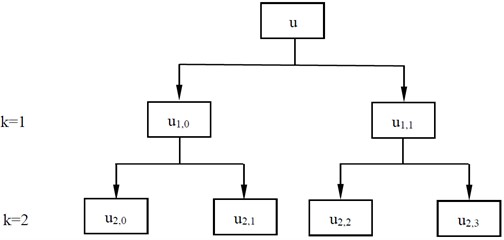(4) The hierarchical component sequence ${u}_{k,e}$ is mapped to $\left[{y}_{1},{y}_{2},...,{y}_{{2}^{k}}\right]$ by the normal cumulative distribution function ${y}_{i}=\frac{1}{\sigma \sqrt{2\pi }}\underset{-\infty }{\overset{{x}_{i}}{\int }}{e}^{\frac{-\left(t-u{\right)}^{2}}{2{\sigma }^{2}}}dt$. ${y}_{i}$ locates in the interval $0~1$.

(5) ${y}_{i}$ is assigned to the integer $1~c$ by the function ${z}_{i}^{c}=round\left(c\mathrm{*}{y}_{i}+0.5\right)$. $c$ is the number of categories. ${z}_{i}^{c}$ is converted to the reconstruction sequence ${z}_{j}^{m,c}$ based on the embedding dimension $m$ and delay parameter $d$:

5

(6) All possible discrete models ${\pi }_{{v}_{1}\cdots {v}_{{c}^{m}}}$ are constructed by using the embedding dimension $m$ and class categories $c$.

(7) ${z}_{j}^{m,c}$ is matched with discrete models ${\pi }_{{v}_{1}\cdots {v}_{{c}^{m}}}$ one by one. Then, Eq. (6) is used to count the frequency of each discrete model ${\pi }_{{v}_{1}\cdots {v}_{{c}^{m}}}$ in the reconstruction sequence ${z}_{j}^{m,c}$:

6
$p\left({\pi }_{{v}_{1}\cdots {v}_{{c}^{m}}}\right)=\frac{Number\left\{j|j\le N-\left(m-1\right)d,{z}_{j}^{m,c}={\pi }_{{v}_{1}\cdots {v}_{{c}^{m}}}\right\}}{N-\left(m-1\right)d}.$

7) Based on the definition of information entropy, the single dispersion entropy is:

7
$e\left(u,m,c,d\right)=-\sum _{\pi =1}^{{c}^{m}}p\left({\pi }_{{v}_{1}\cdots {v}_{{c}^{m}}}\right)\cdot \mathrm{ln}\left(p\left({\pi }_{{v}_{1}\cdots {v}_{{c}^{m}}}\right)\right).$

Finally, the HDE can be expressed as:

8
$HDE=E\left({u}_{k,e},m,c,d\right)=\left[{e}_{1},{e}_{2},\cdots ,{e}_{{2}^{k}}\right].$

## 3. The parameters selection of HDE method

According to the definition of HDE, five parameters need to be set before calculating HDE. Signal length $N$, embedding dimension $m$, number of classes $c$, time delay $d$ and number of decomposition layers $k$. When $k$ is too large, it will affect the calculation efficiency and reduce the points participating in the calculation of each hierarchical component. Otherwise, when the value $k$ is too small, the frequency band division of the original sequence is not detailed enough to obtain enough hierarchical components from low frequency to high frequency. For these reasons, this paper sets $k=\text{3}$. In Generally, time delay $d$ is set 1 to preserve the integrity of feature information in HDE. In order to evaluate the sensitivity of HDE to signal length $N$, this paper calculates the HDE entropy and of 50 groups of white noise and 1/$f$ noise with different length. By calculating the mean and standard deviation at different levels, the degree of node dispersion is judged by coefficient of variation (CV). The coefficient of variation CV is equal to standard deviation / mean. As shown in Fig. 2, the larger the signal length $N$, the higher the stability, and the smaller the error bar. The gap between $N=$ 1024 and $N=$ 4096 is no longer obvious. It can be seen from table 1 that the larger the signal length, the smaller the CV value, and the more stable the calculation of HDE. This paper selects the best signal length $N=$ 1024.

Fig. 2Effect of Signal length Non HDE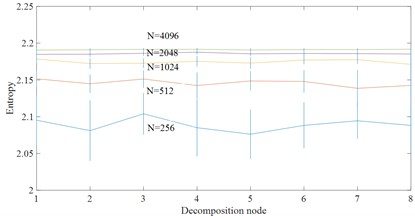a) White noise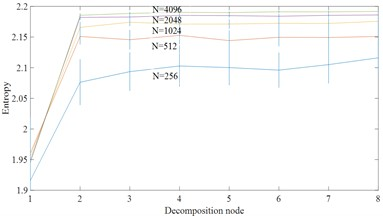b) 1/$f$ noise

Table 1CV values of node 4 for different signal lengths

 Signal length $N$ 256 512 1024 2048 4096 White noise 0.0373 0.0164 0.0064 0.0028 0.0013 1/$f$ noise 0.0321 0.0134 0.007 0.0034 0.0018

From Table 2 and Fig. 3, the CV value of the embedding dimension $m=$ 2 is small and it indicates that HDE value has high stability and small error. In this paper, $m=$ 2 is selected as the best embedding dimension. It can be seen from the CV values of different class numbers in Fig. 4 and Table 3 that the CV value tends to increase with the increase of class number $c$. The coefficient of variation is the smallest and the error rate is the lowest when $c=$ 3. For this reason, $c=$ 3 selected as the best class number.

Fig. 3Effect of embedding dimension m on HDE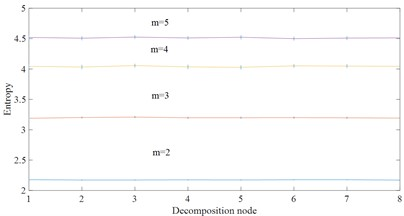a) White noise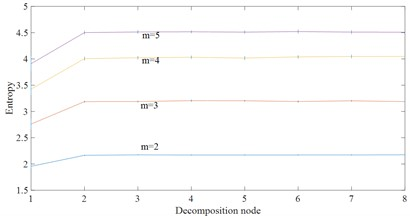b) 1/$f$ noise

Fig. 4Effect of class number c on HDE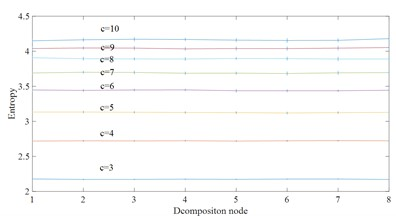a) White noiseb) 1/$f$ noise

Table 2CV values of node 4 for different embedding dimension

 Embedding dimension $m$ 2 3 4 5 White noise 0.0064 0.0112 0.0174 0.0132 1/$f$ noise 0.007 0.0139 0.0153 0.0119

Table 3CV values of node 4 for different classes number c

 Class $c$ 3 4 5 6 7 8 9 10 White noise 0.0064 0.0072 0.0101 0.0092 0.0122 0.0132 0.0128 0.0111 1/$f$ noise 0.007 0.0078 0.0084 0.0092 0.0132 0.0123 0.0142 0.0127

## 4. Experiment

### 4.1. Introduction to the experiment

In this section, the feasibility of the proposed method is verified by the fault data of Case Western Reserve University rolling bearing fault test rig. The fault test rig is presented as in Fig. 5. It consists of a 2 hp Reliance Electric motor driving a shaft on which a torque transducer and encoder are mounted. Torque is applied to the shaft via a dynamometer and electronic control system. For the tests, faults ranging in diameter from 0.007 to 0.028 inch, were seeded on the drive- and fan-end bearings (SKF deep-groove ball bearings: 6205-2RS JEM and 6203-2RS JEM, respectively) of the motor using electro discharge machining (EDM). The faults were seeded on the rolling elements and on the inner and outer races, and each faulty bearing was reinstalled separately on the test rig, which was then run at constant speed for motor loads of 0-3 horsepower (approximate motor speeds of 1797-1720 rpm). During each test, acceleration was measured in the vertical direction on the housing of the drive-end bearing (DE), and in some tests acceleration was also measured in the vertical direction on the fan-end bearing housing (FE) and on the motor supporting base plate (BA). The sample rates used were 12 kHz for some tests and 48 kHz for others, Further details regarding the test set-up can be found at the CWRU Bearing Data Center website.

All the vibration data are taken with the sampling frequency 12 kHz. These data include four different health states of rolling bearings, such as inner race fault, outer race fault, ball fault and normal. Each fault state can be divided into four different damage degrees, 0.007, 0.014, 0.021 and 0.028 inch, respectively.

Fig. 5The rolling bearing fault test rig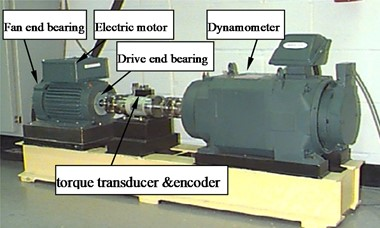In this paper, the vibration signals of the rolling bearing under 10 fault conditions are collected separately. Such as normal, ball fault(0.007), inner race fault(0.007) ,outer race fault(0.007), ball fault(0.014), inner race fault(0.014), outer race fault(0.014), Ball fault(0.021), inner race fault(0.021), outer race fault(0.021). In this experiment, the vibration signal is divided into multiple data sequences, each of which contains 1024 points. Fig. 6 shows the time domain waveform of bearing fault vibration acceleration signal in this experiment. It can be seen from Fig. 6 that relatively obvious fault impact can occur when there are different fault types of rolling bearing.

Fig. 6The vibration signal of four types of rolling bearings faults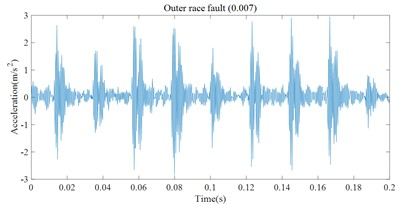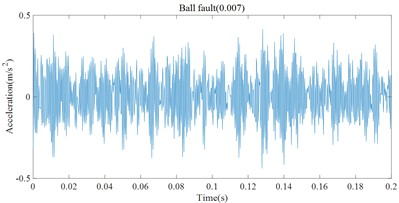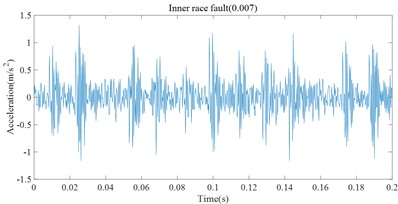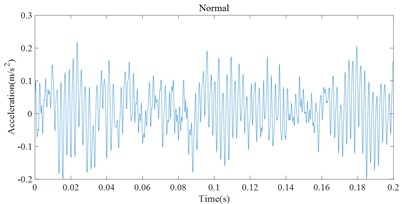For these fault signal, HDE method is used to estimate the entropy for different fault states. In HDE calculation, embedding dimension $m=$ 2, number of classes $c=$ 3, time delay $d=$ 1 and number of decomposition layers $k=$ 3. In this feature vector of this experiment, each fault state consists of 50 samples. Each sample includes 8 entropy attributes. Among them, 25 samples of each fault type are randomly selected to form the training sample set (8×250), and the remaining 25 samples formed the test sample set (8×250). This paper utilizes 90 % of cross validation (10-fold Cross validation) estimation. The random experiment is repeated 10 times. Finally, these samples are input into least square support vector machine (LSSVM)  back propagation neural network (BP) and naive Bayes classifier (Bayes) so as to realize the intelligent recognition of rolling bearing health status. To verify the superiority of the proposed HDE method, MDE and MSE are also used to extract the rolling bearing fault feature vectors at the same time and input them into LSSVM, BP and Bayes method for pattern recognition. The random experiment is also repeated 10 times. The comparison experiment results are presented in Fig. 7 and Table 4. One can see that the three methods all achieve the best classification results in HDE. For example, for the classification method LSSVM, the average accuracy of HDE is 95.2 %, while the average accuracy of MDE is 88.8 %, and the average accuracy of MSE is 87.2 %. For BP and Bayes, the average accuracy of HDE is the highest compared with MDE and MSE. The main reason is that HDE considers both low-frequency components and high-frequency components in the signal, so as to provide more comprehensive and accurate feature information. But, MDE and MSE only considers the low-frequency component of the original sequence and ignores the high-frequency component . Furthermore, the average accuracy of MDE is the higher than MSE. The main reason is that MDE solves the problem of abrupt change in similarity measurement of MSE .

Table 4Comparison of total classification accuracy

 Classification algorithm Classification accuracy HDE MDE MSE Max Min Average Max Min Average Max Min Average LSSVM 96.4 % 94 % 95.2 % 90.4 % 87.6 % 88.8 % 88 % 86.4 % 87.2 % BP 88.4 % 85.2 % 86.4 % 80.4 % 76.8 % 77.2 % 76.8 % 74 % 74.8 % Bayes 88 % 84.8 % 86.8 % 85.6 % 83.2 % 84 % 84.8 % 82.8 % 83.6 %

Moreover, one can compare the accuracy of the three classification methods. For HDE, the average accuracy of LSSVM, BP and bayes is 95.2 %, 86.4 % and 86.8 % respectively. For MDE, the average accuracy of LSSVM, BP and bayes is 88.2 %, 77.8 % and 84 %. For MSE, the average accuracy of LSSVM, BP and Bayes is 87.2 %, 74.8 % and 83.6 %. LSSVM obtain the highest classification accuracy. The main reason is that LSSVM is based on the structured risk minimization theory . The structured risk minimization enables LSSVM to generalize better in the unseen testing samples than neural networks etc. which apply empirical risk minimization.

Fig. 7Fault classification result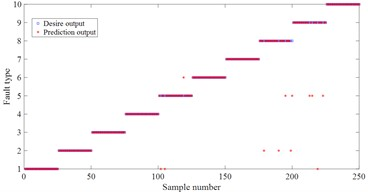a) LSSVM based on HDE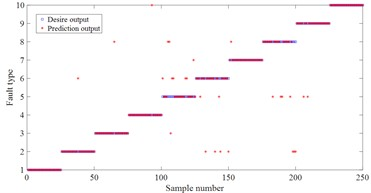b) LSSVM based on MDE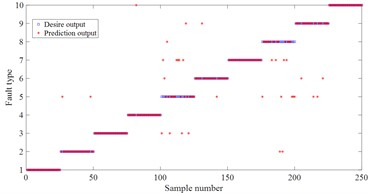c) LSSVM based on MSE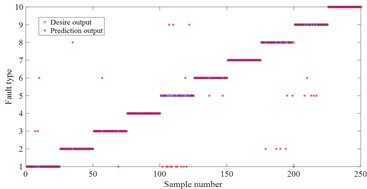d) BP based on HDE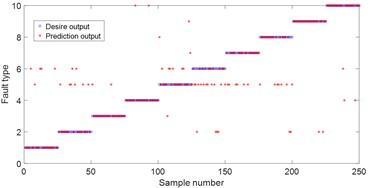e) BP based on MDE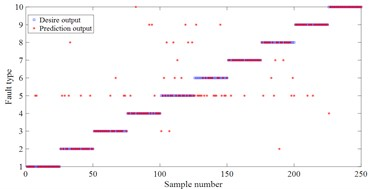f) BP based on MSE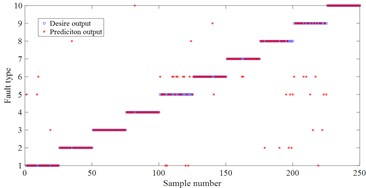g) Bayes based on HDE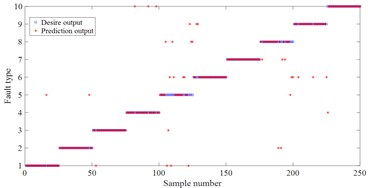h) Bayes based on MDE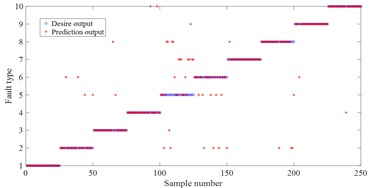i) Bayes based on MSE

## 5. Conclusions

This paper proposes a rolling bearing fault diagnosis method based on the HDE and LSSVM. The effectiveness and practicability of the proposed method are verified by the vibration signals of the rolling bearing. The following conclusions are drawn as:

1) The proposed HDE based on LSSVM can be effectively applied in the fault diagnosis of rolling bearing.

2) HDE can consider the high-frequency and low-frequency components of rolling bearing. On this basis, HDE method can be effectively utilized to measure the complexity of rolling bearing and extract the effect feature vector for different fault types. Experiment results show that the proposed method has higher classification accuracy than the traditional method, MSE, and MDE.

3) Although with higher fault diagnosis accuracy, the new method still in the experimental verification stage based on the rolling bearing experiment system. In terms of this problem, the ability to resist noise should be further improved to make this method more effective in the actual working environment in the future.

#### Acknowledgements

Public Projects of Zhejiang Province (LGF18F030003) and sponsored by K. C. Wong Magna Fund in Ningbo University.